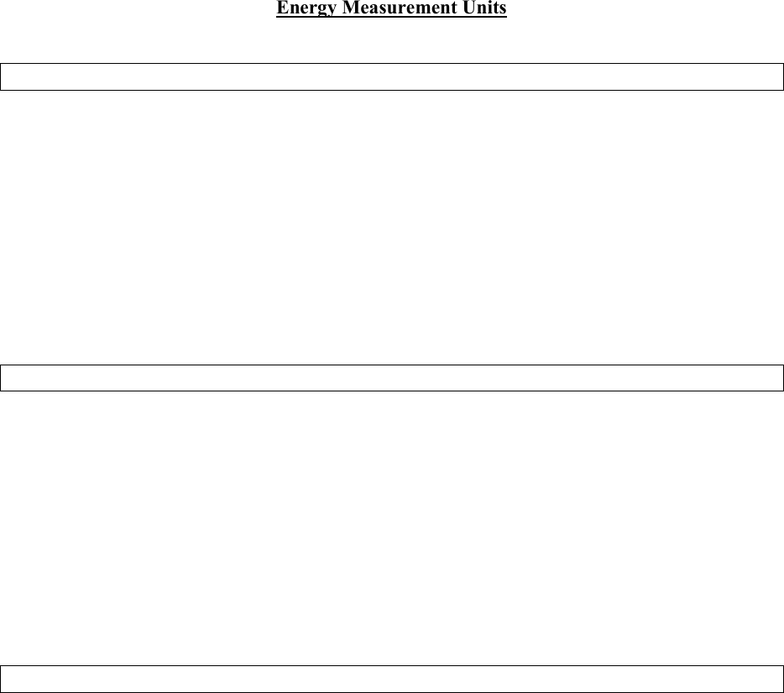# ECON 132 Lecture Notes - Lecture 8: Horsepower, Watt, Kilogram

22 views6 pages
School
UCSD
Department
Economics
Course
ECON 132Energy Units: Conversion and Comparison
Prepared by Genevieve Peters UCSD Economics 132
1 of 6
Energy Measurement Units
Force
Pound-force (lbf) Newton (N)
the force required to give a mass of 1 pound
an acceleration of gravity at the surface of
the Earth
the force required to give a mass of 1 kilogram
an acceleration of 1 meter per second per
second
1 kilogram = 2.2 pounds
1 newton = 0.225 pounds-force
Mechanical Energy
Foot-pound (ft-lb) Joule (J)
mechanical energy required to lift a weight
of 1 pound through a height of 1 foot
the amount of work done when an applied
force of 1 newton moves through a distance
of 1 meter in the direction of the force
1 meter = 3.28 feet
1 foot-pound = 1.36 joules
Power
Horsepower (hp) Watt (W)
550 foot-pounds per second 1 joule per second
1 Horsepower = 746 Watts
1 kilowatt (kW) = 1000 watts
1 megawatt (MW) = 1000 kilowatts
1 gigawatt (GW) = 1000 megawatts
Unlock document

This preview shows pages 1-2 of the document.
Unlock all 6 pages and 3 million more documents.Energy Units: Conversion and Comparison
Prepared by Genevieve Peters UCSD Economics 132
2 of 6
Energy Measurement Units
Heat Energy
British Thermal Unit (Btu) Kilocalorie (kcal)
the quantity of heat energy needed to raise
the temperature of 1 pound of water by 1 F
the quantity of heat energy needed to raise the
temperature of 1 kilogram of water by 1 C.
1 kcal = 4 Btu
1 Btu = 1055 joules
1 kcal = 4220 joules
1F = (1 C x 1.8) + 32
Electrical Energy
Kilowatt-hour (kWh)
the amount of electric energy consumed when an electrical power of 1 kilowatt is maintained for
1 hour.
A 100W lightbulb burning for 10 hours uses 1 kWh of electrical energy.
1 kWh = 3412 Btu
Volume
Oil volumes are typically measured in blue barrels (bbl)
1 bbl = 160 liters
1 bbl 42 U.S. gallons
7 bbls = 1 ton
1 US gallon = 3.78 liters
Coal volumes are typically measured in short tons
1 short ton = 2000 pounds
1 metric ton = 2200 pounds = 1000 kilograms
Natural Gas volumes are typically measured in thousands of cubic feet (Mcf)
1 million cubic feet (MMcf) = 1000 Mcf
1 billion cubic feet (Bcf) = 1,000,000 Mcf
1 trillion cubic feet = 1000 Bcf
Unlock document

This preview shows pages 1-2 of the document.
Unlock all 6 pages and 3 million more documents.

## Document Summary

Pound-force (lbf) the force required to give a mass of 1 pound an acceleration of gravity at the surface of the earth. Newton (n) the force required to give a mass of 1 kilogram an acceleration of 1 meter per second per second. Foot-pound (ft-lb) mechanical energy required to lift a weight of 1 pound through a height of 1 foot. Joule (j) the amount of work done when an applied force of 1 newton moves through a distance of 1 meter in the direction of the force. British thermal unit (btu) the quantity of heat energy needed to raise the temperature of 1 pound of water by 1 f. Kilocalorie (kcal) the quantity of heat energy needed to raise the temperature of 1 kilogram of water by 1 c. 1 f = (1 c x 1. 8) + 32. Kilowatt-hour (kwh) the amount of electric energy consumed when an electrical power of 1 kilowatt is maintained for.